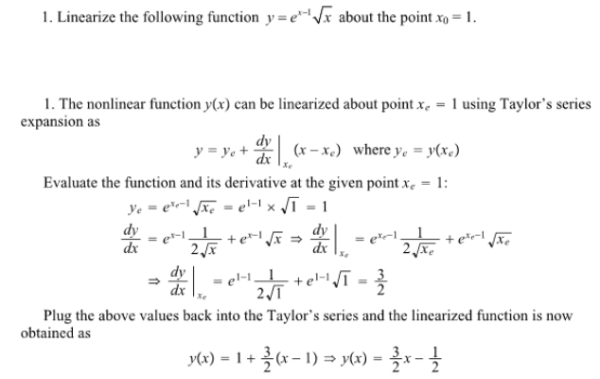Linearize the following function y=e^(x-1)x^1/2 about the point x0=1.Linearize the following function y=e^(x-1)x^1/2 about the point x0=1.

System Dynamics Page 2 dynamics dynamics dynamics dynamics dynamics dynamics dynamics System dynamics Page 3